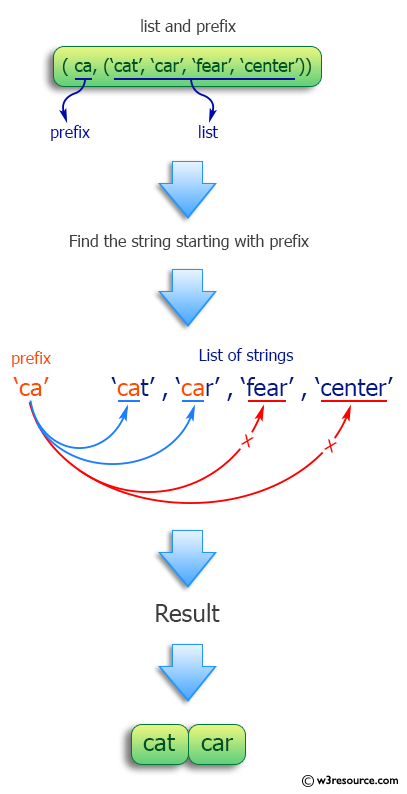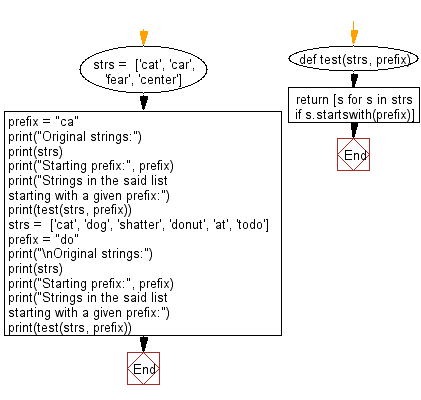﻿ Python: Find the strings in a list, starting with a given prefix - w3resource# Python: Find the strings in a list, starting with a given prefix

## Python Programming Puzzles: Exercise-13 with Solution

Write a Python program to find the strings in a given list, starting with a given prefix.

```Input:
[( ca,('cat', 'car', 'fear', 'center'))]
Output:
['cat', 'car']

Input:
[(do,('cat', 'dog', 'shatter', 'donut', 'at', 'todo'))]
Output:
['dog', 'donut']
```

Pictorial Presentation:Sample Solution:

Python Code:

``````#License: https://bit.ly/3oLErEI

def test(strs, prefix):
return [s for s in strs if s.startswith(prefix)]
strs =  ['cat', 'car', 'fear', 'center']
prefix = "ca"
print("Original strings:")
print(strs)
print("Starting prefix:", prefix)
print("Strings in the said list starting with a given prefix:")
print(test(strs, prefix))
strs =  ['cat', 'dog', 'shatter', 'donut', 'at', 'todo']
prefix = "do"
print("\nOriginal strings:")
print(strs)
print("Starting prefix:", prefix)
print("Strings in the said list starting with a given prefix:")
print(test(strs, prefix))
``````

Sample Output:

```Original strings:
['cat', 'car', 'fear', 'center']
Starting prefix: ca
Strings in the said list starting with a given prefix:
['cat', 'car']

Original strings:
['cat', 'dog', 'shatter', 'donut', 'at', 'todo']
Starting prefix: do
Strings in the said list starting with a given prefix:
['dog', 'donut']
```

Flowchart:## Visualize Python code execution:

The following tool visualize what the computer is doing step-by-step as it executes the said program:

Python Code Editor :

Have another way to solve this solution? Contribute your code (and comments) through Disqus.

What is the difficulty level of this exercise?

Test your Programming skills with w3resource's quiz.

﻿

## Python: Tips of the Day

Clamps num within the inclusive range specified by the boundary values x and y:

Example:

```def tips_clamp_num(num,x,y):
return max(min(num, max(x, y)), min(x, y))
print(tips_clamp_num(2, 4, 6))
print(tips_clamp_num(1, -1, -6))
```

Output:

```4
-1
```